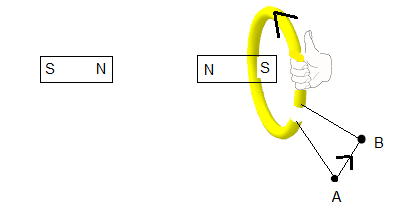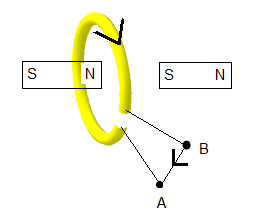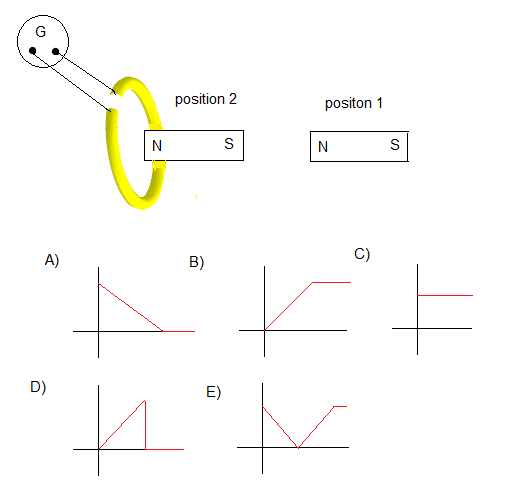# Solutions to SAT Physics Practice Questions on Electromagnetism

Solutions with detailed explanations to sat Physics questions on Electromagnetism.

### Question 1 and its Solution

The primary of a transformer is connected to a source of voltage that has two components: an alternating current (AC) component of 120 volts and a steady direct current (DC) component of 5 volts. The number of turns of the primary is 300 and the that of the secondary is 6000. What is the voltage at the output of the secondary?
A) 2400 volts AC and 100 volts DC
B) 2400 volts AC only
C) 100 volts DC only
D) 2500 volts AC only
E) 2500 volts DC only

Solution - Explanations

Transformers block steady Dc currents and let only AC current through and therefore the output voltage will have an AC component only. The relationship between secondary voltage Vs, the primary voltage Vp, the numbers of turns in the secondary coil Ns and the number of turns in the primary Np is
Vs / Vp = Ns / Np
Vs / 120 = 6000 / 300
gives Vs = 2400 v (AC only)

### Question 2 and its Solution

An electric motor transforms
A) mechanical energy into electrical energy
B) electrical energy into mechanical energy
C) Potential energy into electrical energy
D) A high voltage into a smaller voltage
E) A small voltage into a higher voltage

Solution - Explanations

An electric motor is any device that transforms electrical energy into mechanical energy.

### Question 3 and its Solution

A transformer with an efficiency of 80% is connected to 50-volt alternating current source. The voltage and current in the secondary are 500 Volts and 0.1 Amperes respectively. What is the current in the primary?
A) 0.8 A
B) 1.0 A
C) 1.25 A
D) 1.35 A
E) 1.15 A

Solution - Explanations

The efficiency is the ratio of the power at the secondary of the transformer to the input power at the primary. Hence
80% = ( Is Vs ) / ( Vp Ip )
0.8 = (500 v � 0.1 A) / (50 v � Ip)
Ip = ( 500 v � 0.1 A ) / ( 50 v � 0.8 ) = 1.25 A

### Question 4 and its Solution

A bar of length L slides along electrical wires in a direction perpendicular to a magnetic field B directed out of the page. The bar and part of the wires including the resistor R form a closed circuit. Which of the following will increase the electric current flowing in R?(assume that the resistance due to the wires and the bar is negligible)
(I) decreasing the magnitude of B
(II) moving the bar faster
(III) increasing the length L of the barA) (I)
B) (II) only
C) (III) only
D) (II) and/or (III)
E) (I), (II) and (III)

Solution - Explanations

The sliding bar generates a motional emf E given by
E = L v B , where L is the length of the moving bar, B the magnetic field and v the velocity of the moving bar.
The current i in the circuit id given by
i = E / R = L v B / R
Hence i increases if L or v or B or all are increased.

### Question 5 and its Solution

A permanent magnet is inserted at constant speed inside a loop from left and out of the loop again from the right as shown below. While the magnet is moving from left to right,A) the current in the loop will be from A to B
B) the current in the loop will be from A to B and then from B to A
C) the current in the loop will be from B to A
D) the current in the loop will be from B to A and then from A to B
E) there is no current in the loop

Solution - Explanations

Because of the changing magnetic flux as the permanent magnet moves, an emf is induced in the loop. According to Lenz's law the induced current is such that it will oppose the change producing it. While the magnet is moving from the left, it north pole approaches the loop. Hence the current in the loop should produce magnetic poles that oppose the motion of the magnetic field by creating a north pole to block the magnet. Knowing the magnetic poles of the loop, we use the right hand rule to find the direction of the current.(see figure below). Hence the current is from A to B.Once through the loop, the permanent magnet will have it south pole on the side of the loop and moving away, so the current in the loop must oppose this motion and therefore create a north pole to attract (oppose) the permanent magnet that is trying to move away. Hence the magnetic field in the loop has to change poles and therefore the induced electric field has to change direction. Hence the current is from B to A.(see figure below)### Question 6 and its Solution

A magnet initially stationary is pushed closer to a loop then stopped. Which of the graphs of the current shown below closely represents the variation of the current in the galvanometer detecting the current in the loop?Solution - Explanations

At the start the magnet is stationary and there is no change in the magnetic flux through the loop, therefore no emf is induced and there is no current (= 0). Then as the magnet is pushed the magnetic flux through the loop changes and therefore a current starts building up (increases) in the circuit. As soon as the magnet is stopped the current drops (to 0) because there no change in the magnetic flux. So the graph that closely represent the current in the loop is D

### Question 7 and its Solution

In the figure below the uniform magnetic field B is parallel to the rectangle. What is the magnetic flux of B through the rectangle?A) 0.025 Wb
B) 0
C) 0.004 Wb
D) 250 Wb
E) 0.01 Wb

Solution - Explanations

The flux φ due to a uniform magnetic field B through a planar surface S of area A is given by
φ = B A cos(θ) , where θ is the angle between B and the vector normal to the surface S
In the given diagram B is parallel to the rectangle and therefore perpendicular to any vector normal to the rectangle. Hence in this case θ = 90�
φ = B A cos(90�) = 0

### Question 8 and its Solution

Let Vp, Ip and Np be the voltage, the current and the number of turns of the primary of an electric transformer and Vs, Is and Ns be the voltage, the current and the number of turns of its secondary. If this transformer is not 100% efficient, which of the following must be true?
A) Vp = Vs
B) Vs Is > Vp Ip
C) Vs Is = Vp Ip
D) Ip = Is
E) Vs Is < Vp Ip

Solution - Explanations

The efficiency of a transformer η is defined as
η = Power in the secondary / Power in the primary = ( Vs Is ) / ( V p Ip)
If it is not 100% efficient then η < 100% or η < 1
Hence Vs Is < Vp Ip

### Question 9 and its Solution

Which of the following laws may be used to find the direction of the current induced in a loop close to a moving magnet?
A) Kirchhoff's law
C) Joule's law
D) Lenz's law
E) Ohm's law

Solution - Explanations

According to Lenz's law, the induced current produces a magnetic filed that opposes the changing magnetic field producing it. For example if the magnet is getting closer to the loop, the current produced in the loop must be such that its magnetic field pushes back the approaching magnet. If the magnet is moving away from the loop, the magnetic field due to the induced current must attract the magnet.

### Question 10 and its Solution

Which of the following is true about Lenz's law
(i) It obeys Newton's third Law
(II) It obeys the conservation of energy
(III) It may be used to find direction of induced current
A) (I), (II) and (III)
B) (I) and (II) only
C) (I) and (III) ony
D) (I) only
E) (II) ony

Solution - Explanations

I) Lenz's law is obeys Newton's third (to every action there is a reaction)
II) It obeys the conservation of energy, no energy is destroyed or created in a system where eletromagnetic induction is taking place. There is an exchange of energy between the different parts of the system but the total sum of all the energies is constant.
III) Lenz's law may be used to find the direction of the induced current.